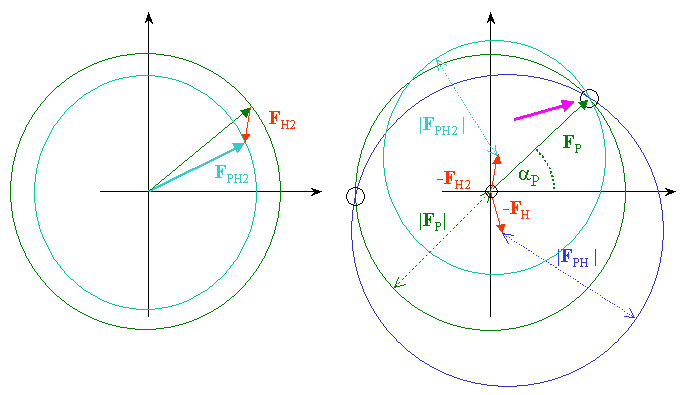#Multiple Isomorphous Replacement (MIR) Phasing

The basic concept of MIR phasing

Isomorphous replacement is the first phasing technique which became available to macromolecular crystallographers. It is based on the fact that the structure factor F(hkl) for a certain reflection hkl is a simple summation of all individual atomic scattering contributions :This allows us to do simple arithmetics with F such as F(p+h) = F(p) + F(h). Let us assume that Fp is the structure factor of a reflection for the protein, and Fph for the same protein now including a heavy atom. Heavy atoms such as transition metals, lanthanides, Uranuim, even noble gasses under pressure, can quite successfully be soaked into crystals through slovent channels and frequently bind to well defined sites in the native protein. Such a protein crystal is then called a heavy atom derivative (crystal). Based under the assumption that the derivative crystal retains its structure (i.e., is in fact isomorphous) we guess that we should be able to derive some information on the structure factor amplitudes for the heavy metal from the differences between the derivative and the native dataset.

Unfortunately, in the absence of phase information we cannot apply the simple subtraction F(h) = F(p+h) - F(p) and end up with the structure factors (and thus positions) of the heavy atom in one step, at least not in the generic non-centrosymmetric case of interest to macromolecular crystallographers. Note also that |Fh| = |Fph| - |Fp| is not correct! This is obvious from the fact that a negative difference in the intensities may well occur - only implying that the phase of Fh is somehow opposite to Fp. We need to separate phase terms from magnitudes in order to do math correctly with a mixture of structure factors F and structure amplitudes |F|.

We need to answer the following questions to attack the problem steps by step :

• What is the formal vector mathematics behind the MIR phasing ?
• How do we actually find the heavy atom positions ?
• How do we get the protein phases and how "good" are they really ?

### Correlation of native and derivative scattering factors

At this point, you may want to briefly review the introduction to vector representation of scattering factors. The following vector diagram (Harker presentation) illustrates the relationship between native and derivative scattering factors. The objective of a phasing experiment is to derive the unknown phase a (p) of each protein reflection Fp.From our the experiment, we know only the magnitudes |Fph| (derivative) and |Fp| (protein) which can be represented in the complex plane as a circle of radius |Fph| and |Fp| , respectively. If we know both the magnitude and the phase of Fh we can draw both circles offset by vector Fh and obtain 2 solutions for possible phase values for Fp (magenta arrows) :The phase and magnitude of Fh can be calculated easily if we know the positions of a heavy metal (we will show in the next chapter how to determine these positions). At this point is is clear that the best phase we can obtain from the 2 solutions is the mean in between the 2 possibilities, and the phase error can be quite large. In real cases, Fh is much shorter that my red vector, and the phase error will be quite large, somewhat below 90 degrees. We realize that on average, SIR (Single Isomorphous Replacement) phases will be better with larger contributions from the heavy metal. More about SIR phases later.

In order to eliminate the phase ambiguity we can prepare a second derivative and repeat the procedure. Provided the heavy atom is not at the same position, we can now obtain a unique solution for a (p), the phase of Fp :We have now, at least in theory, an exact solution for the phase angle of Fp. The theory is based on 2 assumptions : a) ideal isomorphism and b) exact heavy atom positions, neither of which are perfectly met, for practical and experimental reasons in the first case and for theoretical reasons in the second. In our picture it means that the phasing circles may not intersect in exactly in one spot, and another derivative may be necessary to improve the quality of the phases. The method is therefore called MIR, Multiple Isomorphous Replacement. More about MIR phases and their quality later.

What remains at this point is to investigate how we can actually determine the positions of the heavy metal atoms with nothing else but the Structure factor amplitudes of native protein, |Fp|, and of an isomorphous heavy metal derivative, |Fph|. Click her to proceed to calculate some isomorphous derivative Patterson maps. - Working on that....Back to X-ray Tutorial Index
This World Wide Web site conceived and maintained by Bernhard Rupp.
Last revised Dezember 27, 2009 01:40